RootFinding Package Updates - New Features in Maple 2019 - Maplesoft

RootFinding Package Updates in 2019

Description

• The RootFinding:-Isolate command can now be used to isolate the roots of univariate polynomials with arbitrary real coefficients.

Isolating roots of univariate polynomials with real coefficients

• Prior to 2019, RootFinding:-Isolate could only determine the roots of polynomials with rational or float coefficients. This restriction is now lifted for univariate polynomials:

 > with(RootFinding):

 > Isolate(sqrt(2)*x^2 - Pi*x - exp(2));• In particular, Isolate can now be used to find roots of polynomials with algebraic coefficients. We illustrate this in an example where we manually study the real solutions of a bivariate equation system of the form:

 > F := (2*x^2*y - 2*x^2 - 3*x + y^3 - 33*y + 32) * ((x-2)^2 + y^2 + 3):

 > G := (x^2 + y^2 - 23) * (x^2 + y^2 + 2):

• The common roots of both polynomials are the intersections of their corresponding algebraic curves:

 > plots[implicitplot]([F = 0, G = 0], x=-16..16, y=-7..6, color=["Teal", "Red"], gridrefine=2, scaling=constrained, size=[0.7,0.35]);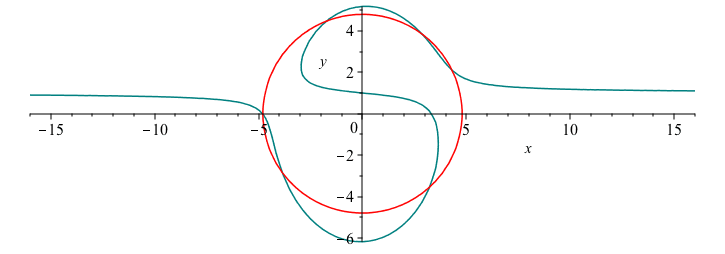• Elimination theory for algebraic equation systems tells us that all x-coordinates of the common solutions are roots of the resultant polynomial ofandwith respect to:

 > R := resultant(F, G, y):

• This resultant is a univariate polynomial with irrational roots, some of which may be complex. The roots are candidate values for the x-coordinate of simultaneous solutions. Note that we store symbolic expressions for the solutions, not just approximations:

 > candidates := sort([RealDomain[solve](R)], key=evalf):

 > evalf(candidates);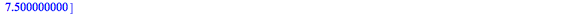• However, some of the candidates might be spurious. We can use the new interface for RootFinding:-Isolate to determine the roots along the fibers ofandwhen we substitute the candidates:

 > a := candidates;> fa := subs(x=a, F):

 > ga := subs(x=a, G):

 > Isolate(fa);> Isolate(ga);• Indeed, there is a common solution close to,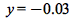:

 > is(RootOf(fa, y, -0.03) = RootOf(ga, y, -0.03));• However, let's look at the candidate at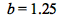:

 > b := candidates;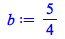> fb := subs(x=b, F):

 > gb := subs(x=b, G):

 > Isolate(fb);> Isolate(gb);• This clearly is a spurious candidate; the roots of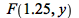andare distinct.

• The example code above can help to filter spurious solutions, but it is not a complete solver for bivariate systems; it allows to filter out suspicious candidates, but does not validate all solutions. Such verification is provided by the multivariate solvers in the RootFinding package:

 > Isolate([F,G], [x,y]);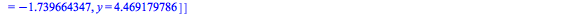• However, the multivariate polynomial solver requires coefficients of type numeric (that is, rationals or floats). Consider the case where we slightly changeby replacingwith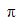in the last term:

 > F := (2*x^2*y - 2*x^2 - 3*x + y^3 - 33*y + 32) * ((x-2)^2 + y^2 + Pi):

 > Isolate([F,G], [x,y]);

 Error, (in RootFinding:-Isolate) polynomials to be solved must have numeric coefficients

• The more naive approach above, while uncertified, will work nevertheless:

 > R := resultant(F, G, y):

 > candidates := sort([RealDomain[solve](R)], key=evalf):

 > evalf(candidates);> a := candidates;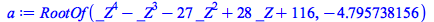> fa := subs(x=a, F):

 > ga := subs(x=a, G):

 > Isolate(fa);> Isolate(ga);> b := candidates;> fb := subs(x=b, F):

 > gb := subs(x=b, G):

 > Isolate(fb);> Isolate(gb);• Finally, we show how the combination of the constraints and output options of RootFinding:-Isolate can provide certified information, and will allow us to programmatically exclude spurious candidates even with irrational coefficients.

 > rts_fb, gb_at_rts_fb := Isolate(fb, constraints=[gb], output=interval):

 > contains_zero := iv -> evalb(iv <= 0 and iv >= 0):

 > seq(contains_zero(rhs(gb_at_rts_fb[i])), i=1..nops(rts_fb));• Indeed,evaluated over all isolating intervals fordoes not contain zero, which confirms thatandhave no common zero at. In contrast,

 > rts_fa, ga_at_rts_fa := Isolate(fa, constraints=[ga], output=interval):

 > seq(contains_zero(rhs(ga_at_rts_fa[i])), i=1..nops(rts_fa));• shows that, evaluated at the isolating intervals for the root of, contains zero. This still does not validate the simultaneous zero of both systems, but is a strong hint. Techniques along these lines can serve to filter candidates numerically before trying time-consuming symbolic simplification and zero-testing, and can be used as cornerstones for complete solvers.

• Note that the aforementioned routines crucially rely on inputs that are served as symbolic expressions rather than approximations:

 > apx := evalf(a);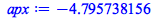> fapx := subs(x=apx, F):

 > gapx := subs(x=apx, G):

 > rts_fapx, gapx_at_rts_fapx := Isolate(fapx, constraints=[gapx], output=interval):

 > seq(contains_zero(rhs(gapx_at_rts_fapx[i])), i=1..nops(rts_fapx));• Even a tiny perturbation of the candidate solution inwill produce distinct roots ofandin. Thus, the direct handling of arbitrary real coefficients is not only convenient, but required for correctness.

Performance improvements for root isolation and root refinement

• The new default algorithm of Isolate also features vastly improved performance for ill-conditioned polynomials with clustered roots. The root finding method eventually converges quadratically to regions containing roots, rather than just linearly. For example, the following class of polynomials has a cluster of roots extremely close to:

 > with(RootFinding):

 > mig := n -> x^n - (nextprime(2^100)*x^2 - 1)^2:

 > time(Isolate(mig(10)));> time(Isolate(mig(10), method=RS));> time(Isolate(mig(50)));> time(Isolate(mig(50), method=RS));> time(Isolate(mig(100)));> time(Isolate(mig(100), method=RS));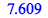> time(Isolate(mig(200)));> timelimit(600, Isolate(mig(200), method=RS));• The same technique allows even more dramatic improvements for root finding requests with high accuracy even on well-conditioned problems:

 > f := add(rand(-1. .. 1.)() * x^i, i=0..100):

 > time(Isolate(f, digits=100));> time(Isolate(f, digits=100, method=RS));> time(Isolate(f, digits=1000));> time(Isolate(f, digits=1000, method=RS));> time(Isolate(f, digits=10000));> timelimit(600, Isolate(f, digits=10000, method=RS));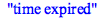Bereit für den nächsten Schritt?

*Die Maple-Evaluation ist für Schüler und Studenten bzw. die private Nutzung zurzeit nicht verfügbar.